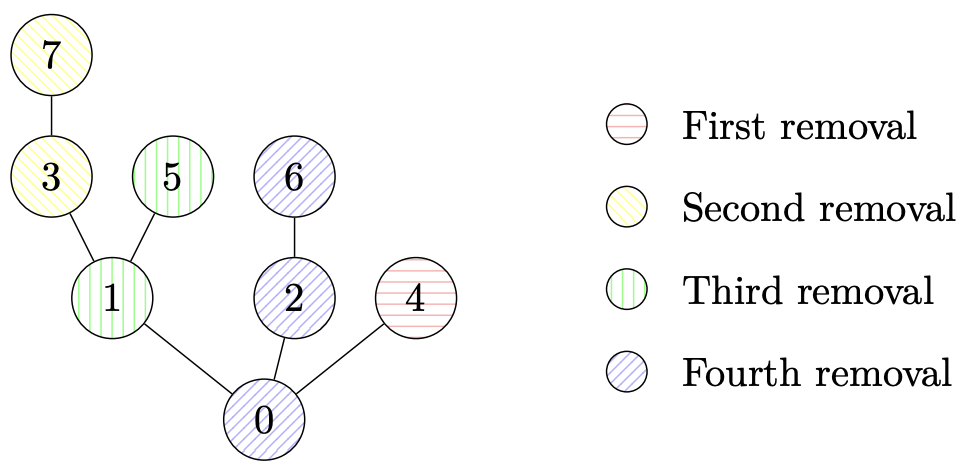시간 제한메모리 제한제출정답맞힌 사람정답 비율
3 초 (추가 시간 없음) 1024 MB222100.000%

## 문제

You can't deny it anymore: the kudzu vines in your garden have grown out of control. Some years ago, you planted a single seedling which you received as a gift from your Mathematics teacher. You vaguely remember her explaining how it grows:

• It started as a single root vertex, labelled $0$.
• Every year, it grows some new vertices and edges: if at the start of the year it has $n$ vertices, then during this year, it will grow a new edge and vertex from each of the $n$ vertices. If an old vertex had index $v$, then the new vertex which grows from it will have index $v+n$.
• It can be shown that after $i$ years, your kudzu plant has exactly $2^i$ vertices, numbered $0$ to $2^i-1$.

Today, it is time to get out your machete knife and remove a number of branches (subtrees) from the kudzu, one by one. You plan on pruning the tree in a fancy shape, which will probably not stay intact for a long time given how fast your kudzu is growing, but at least you promise yourself to keep pruning every year. After deciding which branches you want to cut off today, you call up the Branching And Pruning Company to ask if they can dispose of the plant waste. They want to know exactly how much they need to clean up. You feel like you should be able to compute that, but how?

When a subtree rooted at some vertex $v$ is removed, this means that vertex $v$ will be removed, together with all vertices which have grown from it (and the vertices which have grown from those, and so on). Figure K.1 shows this process for the second sample case.

Given the indices of the roots of the subtrees which you will remove, compute the number of vertices which will be removed for each of these removed subtrees. Since these numbers may be large, you should find them modulo $10^9 + 7$.Figure K.1: The tree of the second sample case. The different colours indicate in which removal the vertices are removed.

## 입력

The input consists of:

• One line containing two integers $a$ ($0 \leq a \leq 10^6$), the age of the tree in years, and $m$ ($1 \leq m \leq 10^5$), the number of subtrees which will be removed.
• $m$ lines, each with an integer $v$ ($0 \leq v \leq 10^9$), the index of a vertex to be removed from the tree. It is guaranteed that $v$ will not yet have been removed.

## 출력

Output $m$ lines. The $i$th line should contain the number of vertices removed in the $i$th removal, modulo $10^9+7$.

## 예제 입력 1

4 1
0


## 예제 출력 1

16


## 예제 입력 2

3 4
4
3
1
0


## 예제 출력 2

1
2
2
3


## 예제 입력 3

5 5
6
3
1
18
2


## 예제 출력 3

4
8
8
1
3


## 예제 입력 4

42 1
0


## 예제 출력 4

46480318


## 출처• 문제를 만든 사람: Reinier Schmiermann